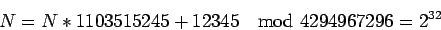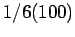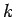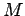Next: The Quadratic Generator Up: Blair's Cryptography Notes Previous: How to play poker   Contents

# Pseudo-random number generators

[This section is based on Blum, Blum, & Shub, A simple unpredictable pseudo-random number generator,'' SIAM J. Computing 15, 364-383.]

Many programs (e. g., simulations, one-time-pads) make use of numbers that are supposed to be random. A genuine source of randomness might be a subroutine that made calls on something like a built-in Geiger counter. We will be concerned with algorithms that produce a sequence of numbers (usually 0's and 1's) which appears random (precise definition will be given later).

A typical example of such an algorithm is the function rand() in the C programming language. Each call updates an internally maintainedusing the formulawith the output given bymod.

I recently wrote a program to roll dice which involved using rand() mod 6. In over 100 calls, it never happened that the same number occurred on two consecutive rolls, even though this should have happened abouttimes! This suggests this particular generator has some problems.13

In this section, we will present random number generators for which it can be proved (given assumptions like (QRA)) that such problems will not occur.

#### Footnotes

... problems.13
Knuth suggests that a better way to obtain a random number between 0 andis to userand(), whereis the maximum value of rand().

SubsectionsNext: The Quadratic Generator Up: Blair's Cryptography Notes Previous: How to play poker   Contents
Translated from LaTeX by Scott Sutherland
2002-12-14